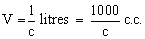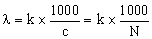# Chemistry Assignment Help with Equivalent Conductance

## 9.5 Equivalent Conductance

More precisely, equivalent conductivity of a solution at a dilution V may be defined as the conductance of all the ions produced from one gram equivalent of the electrolyte dissolved in V. Equivalent conductivity is represented by l or

Mathematically, l = k × V

k = Specific conductivity or electrolytic conductivity

V = Volume of solutions in c.c. containing 1 gm equivalent of the electrolyte.Relationship between equivalent and specific conductivities in terms of concentration Suppose the solution has a concentration of c gram equivalent per litre. Then the volume V containing 1 gram equivalent of the electrolyte will be 1/c litre, i.e.Therefore, the equation becomes### Email Based Assignment Help in Equivalent Conductance

We are the leading online Assignment Help provider. Find answers to all of your doubts regarding the Equivalent Conductance in chemistry. We at assignmenthelp.net provide homework, Assignment Help to the school, college or university level students. Our expert online tutors are available to help you in Equivalent Conductance. Our service is focused on: time delivery, superior quality, creativity and originality.